Question Pool Application of Derivatives

# Application of Derivatives - SAMAGRA Question Pool & Answers | Class 12

Kerala Syllabus SAMAGRA SCERT SAMAGRA Question Pool for Class 12 Maths Application of DerivativesQn 1.
 Let f(x)=a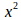+bx+4 be a real function.Where a and b are real numbers. 1.Find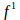(x) 2.If the function attains its minimum value -1 at x=1.Find a and b

Get Free Study Materials + 1 Week Free Trial of BrainsPrep Class 12 Tuition

Qn 2.

Consider the curve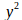= p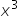+ q,where p and q are real numbers

1.Find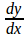at the point (2,3)

2.If y=4x-5 is the tangent to the curve at (2,3) then find the value of p and q

Get Free Study Materials + 1 Week Free Trial of BrainsPrep Class 12 Tuition

Qn 3.

The surface area S=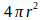of a spherical balloon changes with radius.

1.At what rate does the surface area changes w.r.t the radius

2.Using differentials find approximately how much does the surface area increase when the radius changes from 5cm to 5.2cm

Get Free Study Materials + 1 Week Free Trial of BrainsPrep Class 12 Tuition

Qn 4.

Consider the curve 2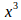-3+27=0

1.Find the points on the curve at which the tangent is parallel to X axis

2.Show that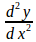=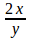-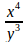,y≠0